# Solution assignment 02 Quadratic functions and graphs

### Assignment 2

Given the function: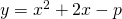1. For which value of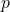does the parabola have no intersection points with the-axis?
2. Calculate the intersection point with the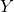-axis.
3. Does the graph has a maximum or a minimum?

### Solution

1. The parabola has no intersection points with the-axis, if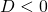, so: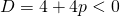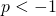2. In this case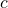in the equation of the parabola equalsand thus the intersection point with the-axi equals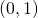.

3. Unregarded the value ofwe have a parabola that opens up (because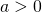) and thus we have a minimum.

0